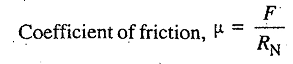# Friction and Its types

Friction and Its types. Static vs. Dynamic friction. Sliding vs. Rolling friction. Limiting friction. Coefficient of Friction.

A force acting in the opposite direction to the motion of the body is called force of friction or simply friction. It is of the following two types

1. Static friction ; and
2. Dynamic friction.

The friction, experienced by a body, when at rest, is known as static friction.

The friction experienced by a body, when in motion, is called dynamic friction. It is also called kinetic friction. It is of the following two types:

(a) Sliding friction ; and
(b) Rolling friction.

The friction, experienced by a body, when it slides over another body, is known as sliding friction.
The friction experienced by a body, when balls or rollers are interposed between the two surfaces, is known as rolling friction.

## Limiting Friction:

The maximum value of frictional force, which comes into play, when a body just begins to slide over the surface of the other body, is known as limiting friction.

## Laws of Static Friction:

Following are the laws of static friction:
1. The force of friction always acts in a direction, opposite to that in which the body tends to move.
2. The magnitude of force of friction is exactly equal to the force, which tends the body to move.
3. The magnitude of the limiting friction bears a constant ratio to the normal reaction between the two surfaces.
4. The force of friction is independent of the area of contact between the two surfaces.
5. The force of friction depends upon the roughness of the surfaces.

## Laws of Dynamic or Kinetic Friction:

Following are the laws of dynamic or kinetic friction :
1. 1. The force of friction always acts in a direction, opposite to that in which the body tends to move.
2. The magnitude of the kinetic friction bears a constant ratio to the normal reaction between the two surfaces.
3. For moderate speeds, the force of friction remains constant. But it decreases slightly with the increase of speed.

## Coefficient of Friction:

It is defined as the ratio of limiting friction (F) to the normal reaction (RN) between the two bodies. It is generally denoted by μ. Mathematically,### You may like these posts:

1.2.3.4.